UGC NET FollowRavi @Eduncle posted an Article
November 21, 2019 • 3:22 pm

# Quick Revision of Walter Model: Theories of Dividend

##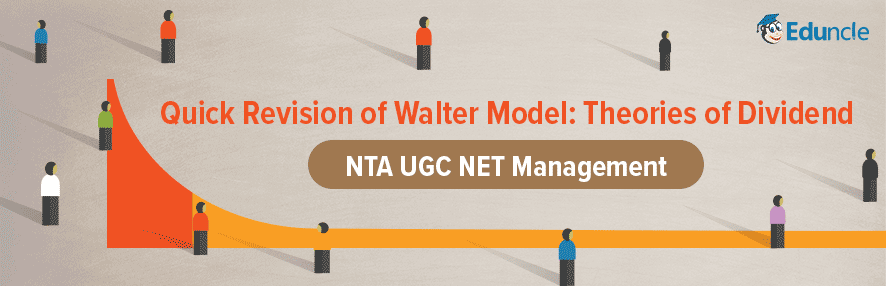Walter Model : Professor James E. Walter has developed a theoretical model which shows the relationship between dividend policies and common stocks prices. According to him the dividend policy of a firm is based on the relationship between the internal rate of return (r) earned by it and the cost of capital or required rate of return (Ke).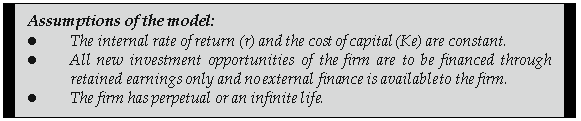As per this model, the investment decisions and dividend decisions of a firm are inter related. A firm should retain its earnings if the return on investment exceeds the cost of capital. Such firms are called Growth Firms (r > Ke). Such firms should have zero pay-out and should re-invest their entire earnings. On the other hand, a firm should distribute its earnings to the shareholder if the internal rate of return is less than the cost of capital (r < Ke). Such firms are called declining firms.

Such firms should distribute the entire profits i.e. 100 per cent pay-out ratio. Firms with internal rate of return equal to the cost of capital (r = Ke) are called Normal Firms. In such firms, the shareholders will be indifferent whether the firm pays dividends or retain the profits.

The formula to determine the market value of share as suggested by Prof. Walter is as under :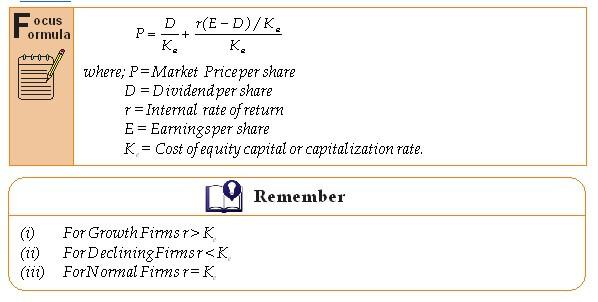Illustration: Santosh Limited earns Rs.5 per share is capitalized at a rate of 10% and has a rate of return on investments of 18%. According the Walter's Formula:

(i) What should be the price per share at 25% dividend pay-out ratio?

(ii) Is this optimum pay-out ratio?

Solution :

(i) Value per share as per Walter formula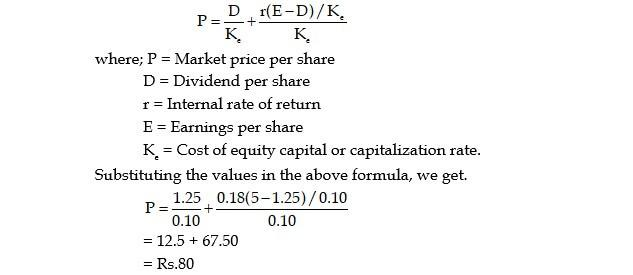(ii) As per above calculation at 25% dividend pay-out, the value of share is Rs.80. But, according to Walter's model, it is not an optimum dividend pay-out because, in such case where internal rate of return is more than the cost of capital (r > Ke), he has suggested zero dividend pay-out.

Illustration: The details regarding three companies are given below :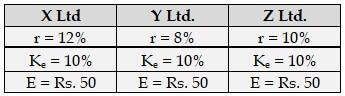Compute the value of an equity share of each of these companies applying Walter's formula when dividend pay-out ratio is (a) 0%, (b) 20%, (c) 40%, (d) 80%, and (e) 100%. Comment on the conclusions drawn.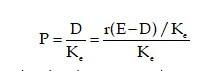Effect of Dividend Policy on Market Price of Shares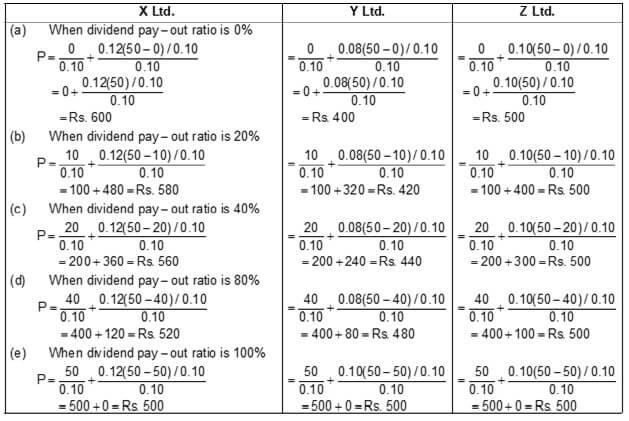### Criticisms of Walter Model

The Walter's model provides a single framework to explain the relationship between dividend policy and value of the firm. If the assumptions underlying the model hold good, the behaviour of the market price of the shares in response to dividend policy of this firm can be explained with the help of this model. But, actually these assumptions are unrealistic and that is why the model is criticised on the following grounds :

(1) Absence of External Financing: Prof. Walter's main assumption that financing of investment proposals only by retained earnings and no external financing is seldom found in real life. Most of the firms meet their financial requirements by loans or issuing new shares.

(2) Stable Internal Rate of Return: The rate of return earned by the firm is never stable. Actually, the rate of return changes with the increase in investments.

(3) Stable Cost of Capital: The assumption of constant cost of capital is also unrealistic, because the risk complexion of the firm is not always uniform. Therefore, cost of capital also changes.

Here we have provided complete information about Walter Model, Theories of Dividend, Effect of Dividend Policy on Market Price of Share and Criticisms of Walter Model.

Still, if you have any query then you can contact us on - "1800-120-1021".

You Might Also Like